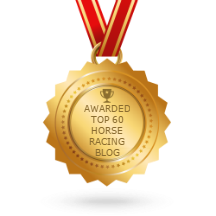# Book %

by Kaz
(UK)Book % Profit

What is the relationship between book % and number of runners in a horse race?

Answer: The book % is the assigning of odds by a bookmaker to each runner in a horse race to at least break even 100% (the round) and then an additional % profit (the overound)

Consider a 5 horse race where odds of 4.0 are assigned to each runner which equates to 25% so that 5 x 25% = 125%

If the bookmaker takes an equal amount on each horse his profit would be 25%

The number of runners would not affect the book % as the bookmaker would always try to achieve the same % profit by adjusting the odds of each horse relative to their chance of winning